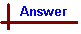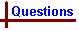For on-line help with this topic, see the chemcal module "Atomic and Nuclear Structure" which deals with the topics: Fundamental concepts; nuclear equations; electromagnetic radiation; wave-particle duality; Bohr model. More advanced topics will be found in the chemcal module "Atoms , Electrons and Orbitals" which deals with the topics: Atomic orbitals - shapes, quantum numbers; subshell structures of atoms and ions. Atomic Structure (General questions) Your feedback on these self-help problems is appreciated. Click here to send an e-mail. Shortcut to Questions Q: 1 2 3 4 5 6 7 8 9 10 1 Give the symbols and ground state electronic orbital configuration of the atoms having the following numbers of electrons: (1) 7 (2) 11 (3) 16 (4) 252 An atom of an element has two electrons in the n=1 shell, eight electrons in the n=2 shell, and five electrons in the n=3 shell. From this information, give for the element (1) its atomic number (2) its approximate atomic weight (3) the total number of s electrons in its atom (4) the total number of d electrons in its atom (5) the name of the element (6) two common oxidation states of the element in its compounds3 List the atomic numbers of the following elements: (1) Li (2) O (3) N (4) Mg (5) Ne (6) Br4 For one atom of the isotope 19E (atomic number 9) give (1) the number of protons (2) the number of electrons (3) the electronic configuration of the atom in the ground state (4) an equation for one typical reaction of the element5 Give the names and symbols (including mass numbers) for the isotopes of hydrogen.6 An energy of 419 kJ mol-1 is required to convert (in the gas phase) potassium atoms to potassium ions and electrons. Given that 1 kJ mol-1 equals 1.67 x 10-21 joule per atom, calculate the longest possible wave length of light which is capable of ionising potassium atoms.7 Calculate (1) the frequency and (2) the energy per quantum for electromagnetic radiation having a wavelength of 580 nm.8 (1) Calculate the wavelength of a photon that has a frequency of 1.20 x 1015 Hz (2) What is the energy of the photon in joules per photon? (3) What is the energy in kJ mol-1 of photons? (4) What is the name usually given to such radiation? (ie what region of the electromagnetic spectrum does it belong to?)9 Calculate for 1.00 MHz broadcast band radio waves: (1) The energy of photons in joules per photon and (2) in kJ mol-1. (3) What is the wavelength of such photons? (4) How does the energy compare with that for a C-C single bond? Would you expect radio waves to be able to produce chemical reactions?10 (Advanced question) Find the energy difference (in kJ mol-1) between the ground and first excited states of the hydrogen atom.Atomic Structure (General answers) 1 The number of electrons in a neutral atom equals the number of protons, so the number of electrons will be equal to the atomic number. (1) Atomic number 7, nitrogen (N): 1s2 2s2 2p3 (2) Atomic number 11, sodium (Na) 1s2 2s2 2p6 3s1 (3) Atomic number 16, sulfur (S): 1s2 2s2 2p6 3s2 3p4 (4) Atomic number 25, manganese (Mn): 1s2 2s2 2p6 3s2 3p6 3d5 4s22 (1) Counting the total number of electrons across all the shells we obtain 15. This must equal the number of protons in the neutral atom, so the atomic number is 15. (2) An element's atomic mass is determined by the number of protons and neutrons, since they contribute practically all mass to the atom. There are approximately equal numbers of protons and neutrons present in atoms, so the approximate mass will be 2 x 15 = 30. (3) (4) From the information given we can work out the element's atomic configuration: Two first shell electrons: 1s2 Eight second shell electrons: 2s2 2p6 Five third shell electrons: 3s2 3p3 Therefore there are a total of six s electrons, and no d electrons. (5) From the atomic number, 15, the element is identified as phosphorus. (6) Phosphorus is a non-metal, and so will form anions in ionic bonds to metals and covalent bonds to non-metals. Gaining 3 electrons (or a share of 3 electrons) fills the 3p orbital making it isoelectronic with Ar. Thus the -III state is common, and shown in the P3- ion, and in covalent molecules such as PH3. Phosphorus can also have more than 8 electrons in its outer level by incorporating the adjacent empty 3d orbitals into the valence level to form 5 covalent bonds, such as in PF5 and PCl5 where the oxidation state of P is +V.3 The atomic number of an element is equal to the number of protons in the nucleus. In a neutral atom the protons (+1 charge) and electrons (-1 charge) are present in equal numbers, so their charges balance. The atomic number of an element is readily determined by consulting a periodic table. (1) Li has an atomic number of 3 (2) O has an atomic number of 8 (3) N has an atomic number of 7 (4) Mg has an atomic number of 12 (5) Ne has an atomic number of 10 (6) Br has an atomic number of 354 (1) The number of protons equals atomic number, which is 9. (2) We assume here that the atom in question is neutral, since no charges have been explicitly mentioned. In a neutral atom the number of electrons equals the number of protons, which is 9. (3) The electronic configuration is found by assigning all available electrons to orbitals, starting with 1s. The configuration for 9 electrons will be 1s2 2s2 2p5. (4) Noting that the outermost orbital (the 2p orbital) is 1 electron short of being filled, the element will assume the -I oxidation state (ie will very readily accept 1 electron) to form stable compounds. Indeed, we can now identify "E" as flourine, which occurs as the F2 molecule. A typical reaction would be the reaction of fluorine with a Group 1 metal such as lithium: 2Li(s) + F2(g) → 2LiF(s)5 hydrogen : 1H (this isotope is also known as protium) deuterium : 2H tritium : 3H6 The energy required to ionise one potassium atom = 419 x 1.67 x 10-21 joule per atom = 7.00 x 10-19 joule per atom. This is the minimum amount of energy required from a single photon of electromagnetic radiation. Converting this energy into a wavelength: E = h c / λ λ = h c / E = (6.626 x 10-34 J s) x (3.00 x 108 m s-1) / (7.00 x 10-19 J) = 2.84 x 10-7 m In the above equation λ (lambda) is wavelength (in metres), h is Planck's constant (6.626 x 10-34 Joule seconds), c is speed of light in vacuum (3.00 x 108 m s-1), and E is energy (in Joules). The minimum wavelength of electromagnetic radiation required to ionise potassium is therefore 284 nm.7 (1) n = c / λ = (3.00 x 108 m s-1) / (580 x 10-9 m) = 5.17 x 1014 Hz Note that frequency is denoted by the symbol n (" nu "), and the units are Hertz which are equivalent to s-1. Also take note of the units used for length which must be in metres to be consistent with energy in Joules. (2) E = h c / λ = (6.626 x 10-34 J s) x (3.00 x 108 m s-1) / (580 x 10-9 m) = 3.43 x 10-19 J8 (1) λ = c / n = (3.00 x 108 m s-1) / (1.20 x 1015 s-1) = 2.50 x 107 m (2) E = h n = (6.626 x 10-34 J s) x (1.20 x 1015 s-1) = 7.95 x 10-19 J (3) There are NA photons in 1 mole = 6.022 x 1023. Therefore the energy of 1 mole of photons = (6.022 x 1023 mol-1) x (7.95 x 10-19 J) = 479 kJ mol-1. (4) A wavelength of 250 nm corresponds to light in the ultraviolet region.9 (1) E = h n = (6.626 x 10-34 J s) x (1.00 x 106 s-1) = 6.626 x 10-28 J per photon. (2) Energy per mole photons = energy per photon x NA = (6.626 x 10-28 J s) x (6.022 x 1023 mol-1) = 3.99 x 10-7 kJ mol-1 (3) λ = h c / E = (6.626 x 10-34 J s) x (3.00 x 108 ms-1) / (6.626 x 10-28 J) = 300 m. (4) The energy of radio waves (3.99 x 10-7 kJ mol-1) is much less than that of a C-C bond (3.48 x 102 kJ mol-1), therefore the waves would not be expected to produce a chemical reaction.10 The Bohr model of the hydrogen atom can be used to predict the energy of various electronic transitions within the hydrogen atom. In this model, electrons are assumed to exist within certain "orbits" (designated by the symbol n) each of which has an associated discrete energy. The energy of an electron in a particular orbit n = E(n) = (-2.18 x 10-18 J) x (1 / n2), for the hydrogen atom only. For the n = 2 → 1 transition, nfinal = 1, and ninitial = 2, so E(n = 2 → 1) = (-2.18 x 10-18 J) x ((1 / 12) - (1 / 22)) = -1.64 x 10-18 J. This is the energy difference per atom, finding the energy in kJ mol-1: E per mole = NA x E(n = 2 → 1) = (6.022 x 1023 mol-1) x (1.64 x 10-18 J) = 988 kJ mol-1.